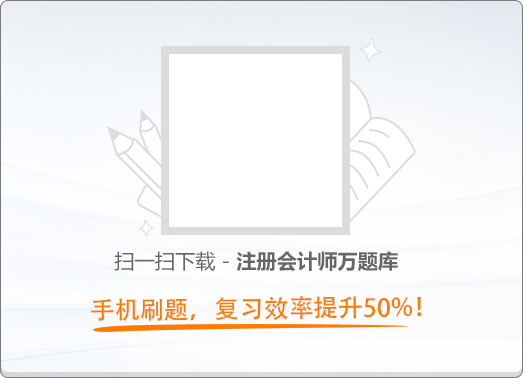150:002014年注册会计师《公司战略与风险管理》真题

1
(单项选择题)

• A.

减损型现金短缺

• B.

增值型现金剩余

• C.

增值型现金短缺

• D.

减损型现金剩余

• A
• B
• C
• D

2
(单项选择题)

• A.

组织协调全面风险管理日常工作

• B.

督导企业风险管理文化的培育

• C.

审议风险管理策略和重大风险管理解决方案

• D.

批准重大决策的风险评估

• A
• B
• C
• D

3
(单项选择题)

• A.

泥石流导致公路中断，使某企业运输途中的设备未能按期送达

• B.

设备零件发生故障，导致某化工企业生产被迫中断

• C.

某高科技企业总经理管理知识缺乏，企业仍处于粗放式管理阶段

• D.

人民币汇率升值导致某外贸企业损失100万元

• A
• B
• C
• D

4
(单项选择题)

• A.

纵轴表示企业销售额增长率

• B.

横轴表示企业在产业中的相对竞争地位

• C.

市场增长率是决定企业产品结构是否合理的外在因素

• D.

波士顿矩阵事实上暗含了一个假设，企业的市场份额与投资回报是正相关的

• A
• B
• C
• D

5
(单项选择题)

• A.

市场风险

• B.

运营风险

• C.

技术风险

• D.

操作风险

• A
• B
• C
• D

6
(单项选择题)

• A.

惩治成本是一种主动的成本费用列支

• B.

损失成本包含给企业带来的潜在经济损失

• C.

纠正成本是风险发生后对企业带来现实危害所付出的成本

• D.

惩治成本是预防成本的追加和补充

• A
• B
• C
• D

7
(单项选择题)

• A.

敏感性分析法

• B.

条件预测法

• C.

情景分析法

• D.

统计推论法

• A
• B
• C
• D

8
(单项选择题)

• A.

企业可以聘请一家会计师事务所为其提供内部控制审计服务和内部控制自我评价服务

• B.

企业内部控制评价工作组应根据现场测试获取的证据，对内部控制缺陷进行最终认定

• C.

评价报告在提交内部控制评价部门前需要得到被评价单位相关责任人签字确认

• D.

企业内部控制评价工作组对内部控制评价报告的真实性负责

• A
• B
• C
• D

9
(单项选择题)

• A.

主要客户、供应商及竞争对手的有关情况

• B.

市场对企业产品或服务的需求

• C.

科技进步、技术创新的有关内容

• D.

企业组织效能和管理现状

• A
• B
• C
• D

10
(单项选择题)

• A.

选择经营范围，发挥协同作用

• B.

确立竞争优势，有效地控制资源的分配和使用

• C.

配置企业内部资源，发挥协同作用

• D.

选择经营范围，合理配置企业经营所需资源

• A
• B
• C
• D

11
(单项选择题)

• A.

集中战略

• B.

混合战略

• C.

差异化战略

• D.

成本领先战略

• A
• B
• C
• D

12
(单项选择题)

• A.

寻求市场

• B.

寻求效率

• C.

寻求资源

• D.

寻求现成资产

• A
• B
• C
• D

13
(单项选择题)

• A.

风险评估

• B.

控制活动

• C.

内部监督

• D.

信息与沟通

• A
• B
• C
• D

14
(单项选择题)

• A.

在编制年度财务报告后，进行资产清查和资产减值测试

• B.

企业应当根据已批准的全面预算编制财务报告

• C.

对财务报告可能产生重大影响的会计政策，按规定权限和程序审批后执行'

• D.

财务报告编制完成后，加盖公章由总会计师签名并盖章后即可对外提供

• A
• B
• C
• D

15
(单项选择题)

• A.

预算管理工作机构设在财会部门

• B.

对投融资项目等重大预算项目，进行密切跟踪和严格监控

• C.

企业董事会审核全面预算草案

• D.

预算管理委员会成员由企业独立董事组成

• A
• B
• C
• D

16
(单项选择题)

• A.

产品开发战略

• B.

混合战略

• C.

产品组合战略

• D.

集中差异化战略

• A
• B
• C
• D

17
(单项选择题)

• A.

一般缺陷

• B.

运行缺陷

• C.

重要缺陷

• D.

设计缺陷

• A
• B
• C
• D

18
(单项选择题)

• A.

有助于预测市场变化或发现战略机会

• B.

有助于寻找产业内的合作伙伴结成战略联盟

• C.

有助于了解产业的进人障碍

• D.

有助于了解产业内企业之间的纵向或横向的联系

• A
• B
• C
• D

19
(单项选择题)

• A.

适宜性标准

• B.

外部性标准

• C.

可行性标准

• D.

可接受性标准

• A
• B
• C
• D

20
(单项选择题)

• A.

两者总是同方向变动

• B.

在劳动密集型产业中，学习经济很小，规模经济却很大

• C.

在资本密集型产业中，规模经济很小，学习经济却很大

• D.

两者交叉地影响产品成本的下降水平

• A
• B
• C
• D

21
(单项选择题)

• A.

财务资源

• B.

企业文化

• C.

商誉

• D.

技术

• A
• B
• C
• D

22
(单项选择题)

• A.

是管理者的权力来源之一

• B.

主要存在于正式组织中

• C.

是管理者在管理层次中的体现

• D.

是管理者对资源的控制的体现

• A
• B
• C
• D

23
(单项选择题)

• A.

波士顿矩阵

• B.

成功关键因素分析

• C.

SWOT分析

• D.

通用矩阵

• A
• B
• C
• D

24
(单项选择题)

• A.

快速反应能力

• B.

成长能力

• C.

适应变化的能力

• D.

持久力

• A
• B
• C
• D